## 8.4 Constructing confidence intervals

Recall that the process of resampling with replacement we performed by hand in Section 8.1 and virtually in Section 8.2 is known as bootstrapping. The term bootstrapping originates in the expression of “pulling oneself up by their bootstraps,” meaning to “succeed only by one’s own efforts or abilities.”

From a statistical perspective, bootstrapping alludes to succeeding in being able to study the effects of sampling variation on estimates from the “effort” of a single sample. Or more precisely, it refers to constructing an approximation to the sampling distribution using only one sample.

To perform this resampling with replacement virtually in Section 8.2, we used the rep_sample_n() function, making sure that the size of the resamples matched the original sample size of 50. In this section, we’ll build off these ideas to construct confidence intervals using a new package: the infer package for “tidy” and transparent statistical inference.

### 8.4.1 Original workflow

Recall that in Section 8.2, we virtually performed bootstrap resampling with replacement to construct bootstrap distributions. Such distributions are approximations to the sampling distributions we saw in Chapter 7, but are constructed using only a single sample. Let’s revisit the original workflow using the %>% pipe operator.

First, we used the rep_sample_n() function to resample size = 50 pennies with replacement from the original sample of 50 pennies in pennies_sample by setting replace = TRUE. Furthermore, we repeated this resampling 1000 times by setting reps = 1000:

pennies_sample %>%
rep_sample_n(size = 50, replace = TRUE, reps = 1000)

Second, since for each of our 1000 resamples of size 50, we wanted to compute a separate sample mean, we used the dplyr verb group_by() to group observations/rows together by the replicate variable…

pennies_sample %>%
rep_sample_n(size = 50, replace = TRUE, reps = 1000) %>%
group_by(replicate) 

… followed by using summarize() to compute the sample mean() year for each replicate group:

pennies_sample %>%
rep_sample_n(size = 50, replace = TRUE, reps = 1000) %>%
group_by(replicate) %>%
summarize(mean_year = mean(year))

For this simple case, we can get by with using the rep_sample_n() function and a couple of dplyr verbs to construct the bootstrap distribution. However, using only dplyr verbs only provides us with a limited set of tools. For more complicated situations, we’ll need a little more firepower. Let’s repeat this using the infer package.

### 8.4.2infer package workflow

The infer package is an R package for statistical inference. It makes efficient use of the %>% pipe operator we introduced in Section 3.1 to spell out the sequence of steps necessary to perform statistical inference in a “tidy” and transparent fashion. Furthermore, just as the dplyr package provides functions with verb-like names to perform data wrangling, the infer package provides functions with intuitive verb-like names to perform statistical inference.

Let’s go back to our pennies. Previously, we computed the value of the sample mean $$\overline{x}$$ using the dplyr function summarize():

pennies_sample %>%
summarize(stat = mean(year))

We’ll see that we can also do this using infer functions specify() and calculate():

pennies_sample %>%
specify(response = year) %>%
calculate(stat = "mean")

You might be asking yourself: “Isn’t the infer code longer? Why would I use that code?”. While not immediately apparent, you’ll see that there are three chief benefits to the infer workflow as opposed to the dplyr workflow.

First, the infer verb names better align with the overall resampling framework you need to understand to construct confidence intervals and to conduct hypothesis tests (in Chapter 9). We’ll see flowchart diagrams of this framework in the upcoming Figure 8.23 and in Chapter 9 with Figure 9.14.

Second, you can jump back and forth seamlessly between confidence intervals and hypothesis testing with minimal changes to your code. This will become apparent in Subsection 9.3.2 when we’ll compare the infer code for both of these inferential methods.

Third, the infer workflow is much simpler for conducting inference when you have more than one variable. We’ll see two such situations. We’ll first see situations of two-sample inference where the sample data is collected from two groups, such as in Section 8.6 where we study the contagiousness of yawning and in Section 9.1 where we compare promotion rates of two groups at banks in the 1970s. Then in Section 10.4, we’ll see situations of inference for regression using the regression models you fit in Chapter 5.

Let’s now illustrate the sequence of verbs necessary to construct a confidence interval for $$\mu$$, the population mean year of minting of all US pennies in 2019.

#### 1. specify variables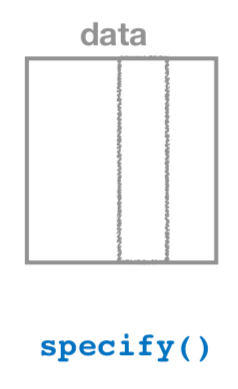FIGURE 8.18: Diagram of the specify() verb.

As shown in Figure 8.18, the specify() function is used to choose which variables in a data frame will be the focus of our statistical inference. We do this by specifying the response argument. For example, in our pennies_sample data frame of the 50 pennies sampled from the bank, the variable of interest is year:

pennies_sample %>%
specify(response = year)
Response: year (numeric)
# A tibble: 50 x 1
year
<dbl>
1  2002
2  1986
3  2017
4  1988
5  2008
6  1983
7  2008
8  1996
9  2004
10  2000
# … with 40 more rows

Notice how the data itself doesn’t change, but the Response: year (numeric) meta-data does. This is similar to how the group_by() verb from dplyr doesn’t change the data, but only adds “grouping” meta-data, as we saw in Section 3.4.

We can also specify which variables will be the focus of our statistical inference using a formula = y ~ x. This is the same formula notation you saw in Chapters 5 and 6 on regression models: the response variable y is separated from the explanatory variable x by a ~ (“tilde”). The following use of specify() with the formula argument yields the same result seen previously:

pennies_sample %>%
specify(formula = year ~ NULL)

Since in the case of pennies we only have a response variable and no explanatory variable of interest, we set the x on the right-hand side of the ~ to be NULL.

While in the case of the pennies either specification works just fine, we’ll see examples later on where the formula specification is simpler. In particular, this comes up in the upcoming Section 8.6 on comparing two proportions and Section 10.4 on inference for regression.

#### 2. generate replicates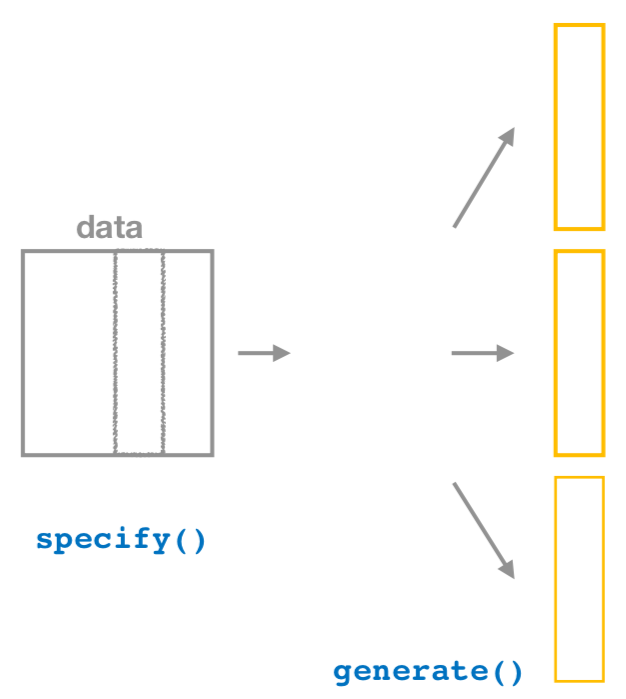FIGURE 8.19: Diagram of generate() replicates.

After we specify() the variables of interest, we pipe the results into the generate() function to generate replicates. Figure 8.19 shows how this is combined with specify() to start the pipeline. In other words, repeat the resampling process a large number of times. Recall in Sections 8.2.2 and 8.2.3 we did this 35 and 1000 times.

The generate() function’s first argument is reps, which sets the number of replicates we would like to generate. Since we want to resample the 50 pennies in pennies_sample with replacement 1000 times, we set reps = 1000. The second argument type determines the type of computer simulation we’d like to perform. We set this to type = "bootstrap" indicating that we want to perform bootstrap resampling. You’ll see different options for type in Chapter 9.

pennies_sample %>%
specify(response = year) %>%
generate(reps = 1000, type = "bootstrap")
Response: year (numeric)
# A tibble: 50,000 x 2
# Groups:   replicate [1,000]
replicate  year
<int> <dbl>
1         1  1981
2         1  1988
3         1  2006
4         1  2016
5         1  2002
6         1  1985
7         1  1979
8         1  2000
9         1  2006
10         1  2016
# … with 49,990 more rows

Observe that the resulting data frame has 50,000 rows. This is because we performed resampling of 50 pennies with replacement 1000 times and 50,000 = 50 $$\cdot$$ 1000.

The variable replicate indicates which resample each row belongs to. So it has the value 1 50 times, the value 2 50 times, all the way through to the value 1000 50 times. The default value of the type argument is "bootstrap" in this scenario, so if the last line was written as generate(reps = 1000), we’d obtain the same results.

Comparing with original workflow: Note that the steps of the infer workflow so far produce the same results as the original workflow using the rep_sample_n() function we saw earlier. In other words, the following two code chunks produce similar results:

# infer workflow:                   # Original workflow:
pennies_sample %>%                  pennies_sample %>%
specify(response = year) %>%        rep_sample_n(size = 50, replace = TRUE,
generate(reps = 1000)                            reps = 1000)              

#### 3. calculate summary statistics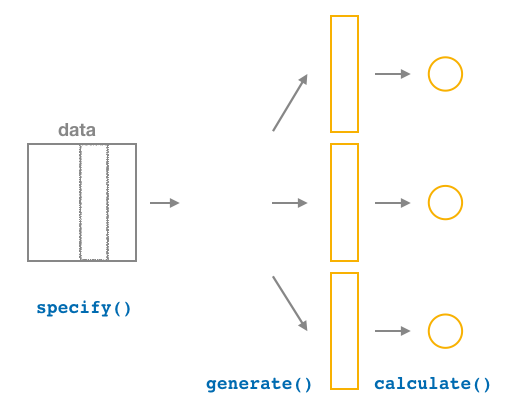FIGURE 8.20: Diagram of calculate() summary statistics.

After we generate() many replicates of bootstrap resampling with replacement, we next want to summarize each of the 1000 resamples of size 50 to a single sample statistic value. As seen in the diagram, the calculate() function does this.

In our case, we want to calculate the mean year for each bootstrap resample of size 50. To do so, we set the stat argument to "mean". You can also set the stat argument to a variety of other common summary statistics, like "median", "sum", "sd" (standard deviation), and "prop" (proportion). To see a list of all possible summary statistics you can use, type ?calculate and read the help file.

Let’s save the result in a data frame called bootstrap_distribution and explore its contents:

bootstrap_distribution <- pennies_sample %>%
specify(response = year) %>%
generate(reps = 1000) %>%
calculate(stat = "mean")
bootstrap_distribution
# A tibble: 1,000 x 2
replicate    stat
<int>   <dbl>
1         1 1995.7
2         2 1994.04
3         3 1993.62
4         4 1994.5
5         5 1994.08
6         6 1993.6
7         7 1995.26
8         8 1996.64
9         9 1994.3
10        10 1995.94
# … with 990 more rows

Observe that the resulting data frame has 1000 rows and 2 columns corresponding to the 1000 replicate values. It also has the mean year for each bootstrap resample saved in the variable stat.

Comparing with original workflow: You may have recognized at this point that the calculate() step in the infer workflow produces the same output as the group_by() %>% summarize() steps in the original workflow.

# infer workflow:                   # Original workflow:
pennies_sample %>%                  pennies_sample %>%
specify(response = year) %>%        rep_sample_n(size = 50, replace = TRUE,
generate(reps = 1000) %>%                        reps = 1000) %>%
calculate(stat = "mean")            group_by(replicate) %>%
summarize(stat = mean(year))

#### 4. visualize the results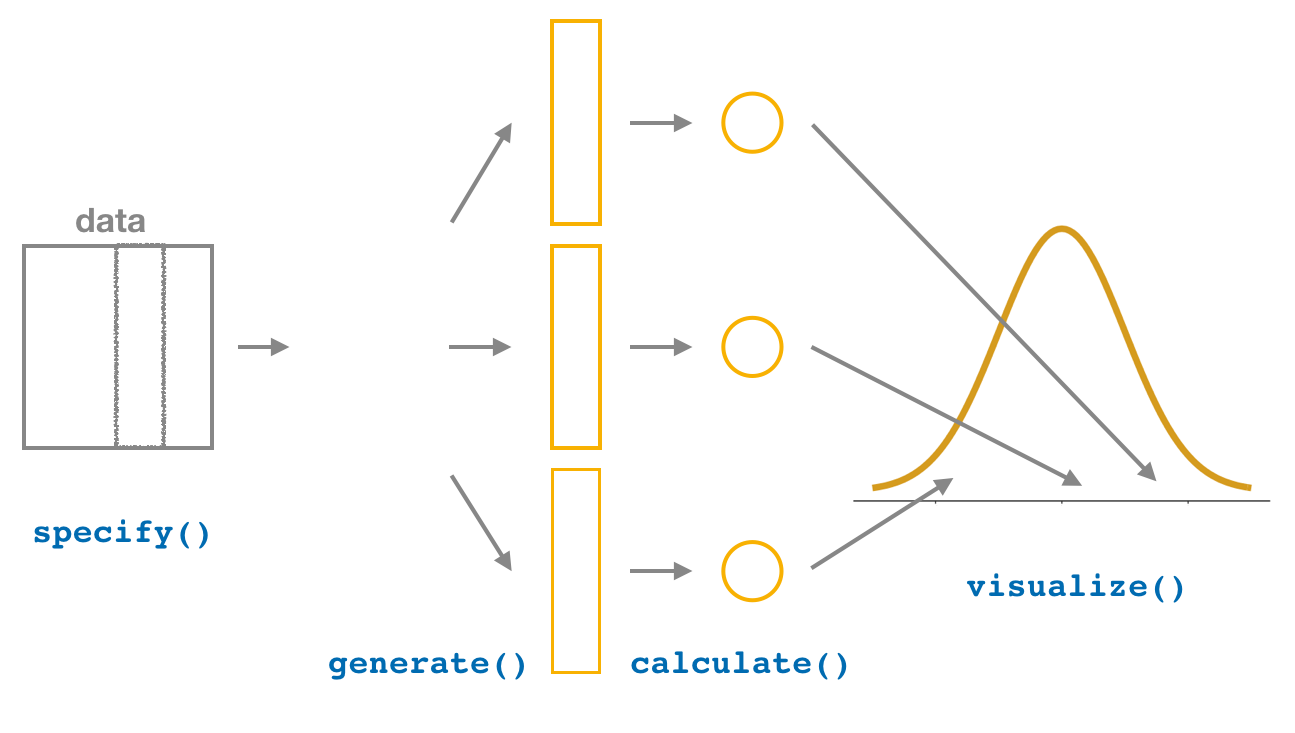FIGURE 8.21: Diagram of visualize() results.

The visualize() verb provides a quick way to visualize the bootstrap distribution as a histogram of the numerical stat variable’s values. The pipeline of the main infer verbs used for exploring bootstrap distribution results is shown in Figure 8.21.

visualize(bootstrap_distribution)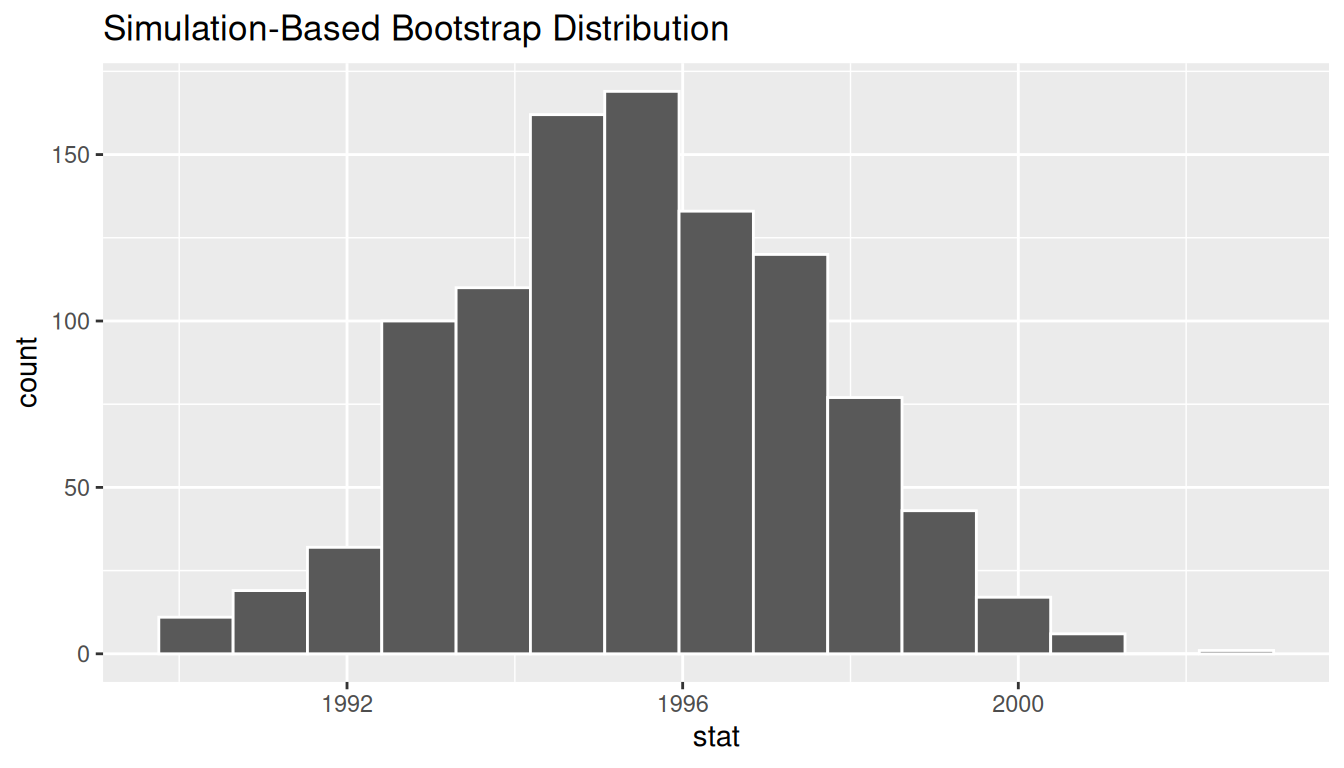FIGURE 8.22: Bootstrap distribution.

Comparing with original workflow: In fact, visualize() is a wrapper function for the ggplot() function that uses a geom_histogram() layer. Recall that we illustrated the concept of a wrapper function in Figure 5.5 in Subsection 5.1.2.

# infer workflow:                    # Original workflow:
visualize(bootstrap_distribution)    ggplot(bootstrap_distribution,
aes(x = stat)) +
geom_histogram()

The visualize() function can take many other arguments which we’ll see momentarily to customize the plot further. It also works with helper functions to do the shading of the histogram values corresponding to the confidence interval values.

Let’s recap the steps of the infer workflow for constructing a bootstrap distribution and then visualizing it in Figure 8.23.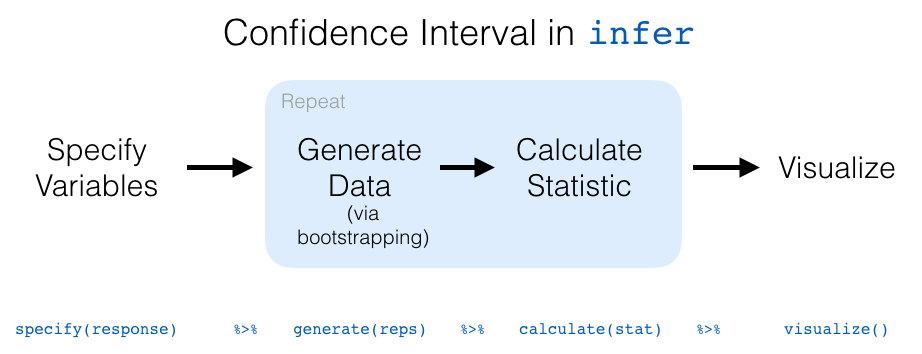FIGURE 8.23: infer package workflow for confidence intervals.

Recall how we introduced two different methods for constructing 95% confidence intervals for an unknown population parameter in Section 8.3: the percentile method and the standard error method. Let’s now check out the infer package code that explicitly constructs these. There are also some additional neat functions to visualize the resulting confidence intervals built-in to the infer package!

### 8.4.3 Percentile method with infer

Recall the percentile method for constructing 95% confidence intervals we introduced in Subsection 8.3.1. This method sets the lower endpoint of the confidence interval at the 2.5th percentile of the bootstrap distribution and similarly sets the upper endpoint at the 97.5th percentile. The resulting interval captures the middle 95% of the values of the sample mean in the bootstrap distribution.

We can compute the 95% confidence interval by piping bootstrap_distribution into the get_confidence_interval() function from the infer package, with the confidence level set to 0.95 and the confidence interval type to be "percentile". Let’s save the results in percentile_ci.

percentile_ci <- bootstrap_distribution %>%
get_confidence_interval(level = 0.95, type = "percentile")
percentile_ci
# A tibble: 1 x 2
2.5% 97.5%
<dbl>   <dbl>
1 1991.24 1999.42

Alternatively, we can visualize the interval (1991.24, 1999.42) by piping the bootstrap_distribution data frame into the visualize() function and adding a shade_confidence_interval() layer. We set the endpoints argument to be percentile_ci.

visualize(bootstrap_distribution) +
shade_confidence_interval(endpoints = percentile_ci)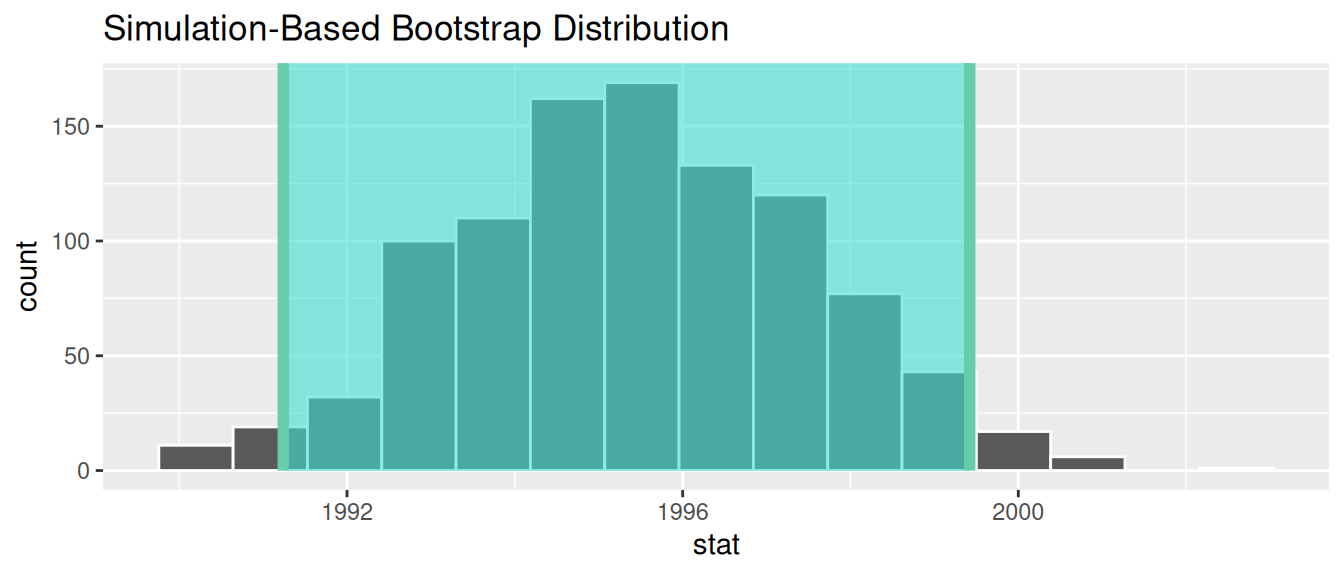FIGURE 8.24: Percentile method 95% confidence interval shaded corresponding to potential values.

Observe in Figure 8.24 that 95% of the sample means stored in the stat variable in bootstrap_distribution fall between the two endpoints marked with the darker lines, with 2.5% of the sample means to the left of the shaded area and 2.5% of the sample means to the right. You also have the option to change the colors of the shading using the color and fill arguments.

You can also use the shorter named function shade_ci() and the results will be the same. This is for folks who don’t want to type out all of confidence_interval and prefer to type out ci instead. Try out the following code!

visualize(bootstrap_distribution) +
shade_ci(endpoints = percentile_ci, color = "hotpink", fill = "khaki")

### 8.4.4 Standard error method with infer

Recall the standard error method for constructing 95% confidence intervals we introduced in Subsection 8.3.2. For any distribution that is normally shaped, roughly 95% of the values lie within two standard deviations of the mean. In the case of the bootstrap distribution, the standard deviation has a special name: the standard error.

So in our case, 95% of values of the bootstrap distribution will lie within $$\pm 1.96$$ standard errors of $$\overline{x}$$. Thus, a 95% confidence interval is

$\overline{x} \pm 1.96 \cdot SE = (\overline{x} - 1.96 \cdot SE, \, \overline{x} + 1.96 \cdot SE).$

Computation of the 95% confidence interval can once again be done by piping the bootstrap_distribution data frame we created into the get_confidence_interval() function. However, this time we set the first type argument to be "se". Second, we must specify the point_estimate argument in order to set the center of the confidence interval. We set this to be the sample mean of the original sample of 50 pennies of 1995.44 we saved in x_bar earlier.

standard_error_ci <- bootstrap_distribution %>%
get_confidence_interval(type = "se", point_estimate = x_bar)
standard_error_ci
# A tibble: 1 x 2
lower   upper
<dbl>   <dbl>
1 1991.35 1999.53

If we would like to visualize the interval (1991.35, 1999.53), we can once again pipe the bootstrap_distribution data frame into the visualize() function and add a shade_confidence_interval() layer to our plot. We set the endpoints argument to be standard_error_ci. The resulting standard-error method based on a 95% confidence interval for $$\mu$$ can be seen in Figure 8.25.

visualize(bootstrap_distribution) +
shade_confidence_interval(endpoints = standard_error_ci)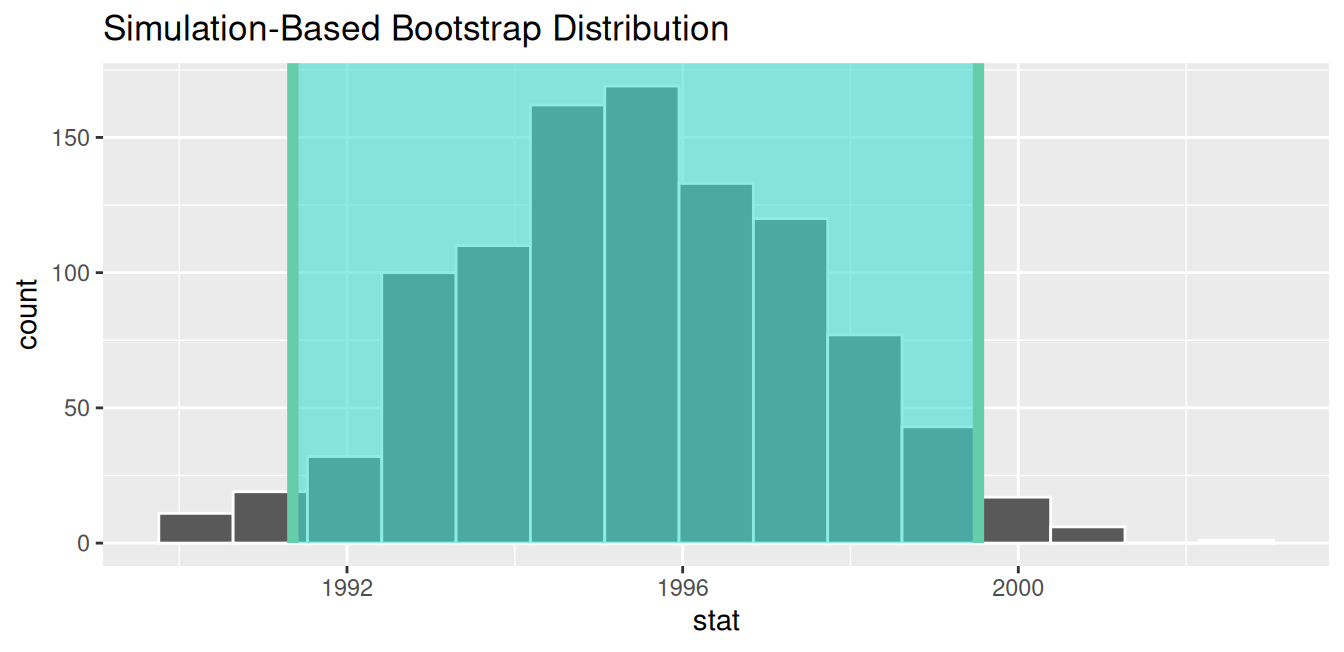FIGURE 8.25: Standard-error-method 95% confidence interval.

As noted in Section 8.3, both methods produce similar confidence intervals:

• Percentile method: (1991.24, 1999.42)
• Standard error method: (1991.35, 1999.53)

Learning check

(LC8.5) Construct a 95% confidence interval for the median year of minting of all US pennies. Use the percentile method and, if appropriate, then use the standard-error method.